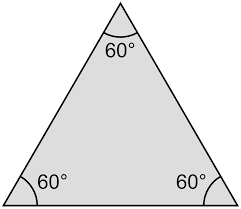QuestionAnswers

# State the name of a regular polygon of 3 sides.A. Equilateral TriangleB. Isosceles TriangleC. Scalene TriangleD. None of theseVerified
128.1k+ views
Hint: First, we will break the given data into different terms and write the meanings of those and then evaluate by the data what kind of triangle we get or if we get some other closed figure, then the answer will be D.

First of all we have the term regular polygon.
We will first get to know what do we mean by regular polygon.
A regular polygon is equiangular and equilateral.
Equiangular means all the angles in the polygon have the same measure and equilateral means that all the sides of the polygon are equal.
So, we now have a figure with all equal angles and all equal sides.
We also have 3 sides.
We know that the sum of exterior angles of a regular polygon is always 360$^ \circ$and the measure of each angle is $\dfrac{{{{360}^ \circ }}}{n}$, where n is the number of sides of the regular polygon.
We have n = 3. So, measure of each angle will be $\dfrac{{{{360}^ \circ }}}{n} = \dfrac{{{{360}^ \circ }}}{3} = {120^ \circ }$.
We now know that measure of exterior angle = sum of opposite interior angles.
So, if the interior angle is a$^ \circ$, then 2a = 120$^ \circ$ that is a = 60$^ \circ$.
Now, we have a polygon with all equal sides and all angles with measure of 60$^ \circ$.
Since, only the equilateral triangle has all the three sides equal and all the angles to be measuring 60$^ \circ$.
So, we have an equilateral triangle.So, the correct answer is “Option A”.

Note: We have used a lot of facts in this solution. We must carefully remember all the facts that are:
Fact 1: A regular polygon is equiangular and equilateral. Equiangular means all the angles in the polygon have the same measure and equilateral means that all the sides of the polygon are equal.
Fact 2: Sum of exterior angles of a regular polygon is always 360$^ \circ$and the measure of each angle is $\dfrac{{{{360}^ \circ }}}{n}$, where n is the number of sides of the regular polygon.
Fact 3: Measure of exterior angle = sum of opposite interior angles.